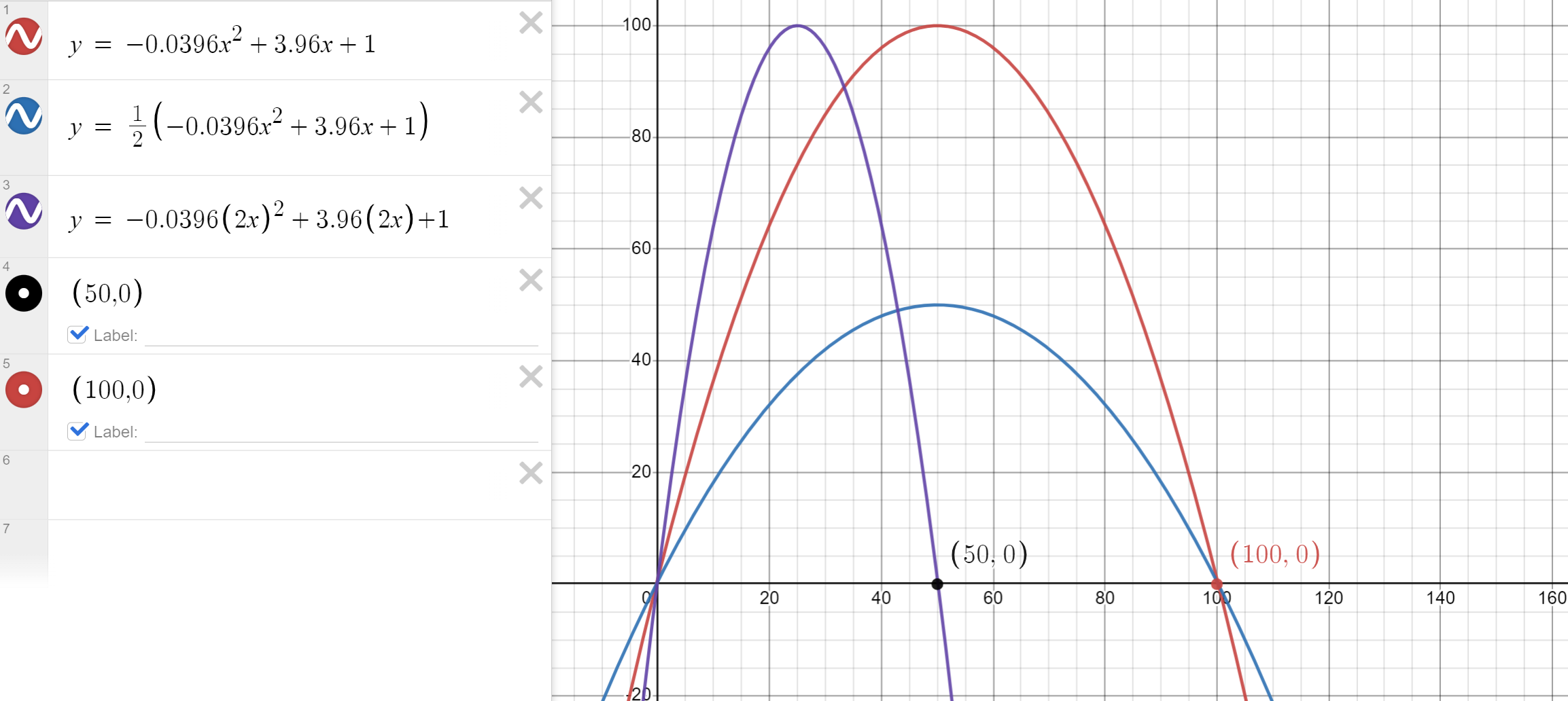# Rng System With Luck increase

Not sure how i would make a rng type system that increases with luck.
but also if you have enough luck it would decrease the chances of the more common things

for example
with 1 luck it would look something like this

``````local Luck = 1

local TableOfRaritys = {
["CommonItem"] = 100,
["UncommonItem"] = 80,
["RareItem"] = 50,
["EpicItem"] = 20,
["LegendaryItem"] = 5,
}
``````

With 10 luck

``````local Luck = 10

local TableOfRaritys = {
["CommonItem"] = 80,
["UncommonItem"] = 100,
["RareItem"] = 80,
["EpicItem"] = 40,
["LegendaryItem"] = 15,
}
``````

and with 100 luck

``````local Luck = 100

local TableOfRaritys = {
["CommonItem"] = 1, -- or 0
["UncommonItem"] = 20,
["RareItem"] = 70,
["EpicItem"] = 100,
["LegendaryItem"] = 55,
}
``````

Sorry if this is vague, i couldn’t really wrap my head around how to explain it

2 Likes

Could this be what you’re looking for?

1 Like

This is apart of it but not it fully, this will help though

1 Like

Could still use some help on thisYou can use equations to weight your items. Exponential functions might be a good idea.
If I were you I’d just play around on a graphing calculator, here’s one I made for `["CommonItem"]`

ion got this but ill try to brute-force italso what if it was for any other item?
would it need to go up then down? like with `["UncommonItem"]`

Here’s the function I personally use:

``````function getRandomItem(Table)
local total = 0
for i, v in pairs(Table) do
total += v
end
local r = RNG:NextNumber(0, total)
for item, v in pairs(Table) do
return item
end
end
return 0
end
``````

This function gets a dictionary with the result as the key, and the chance as the number. For example:

``````local Items = {
Common = 100;
Uncommon = 30;
Rare = 10;
Epic = 2;
Legendary = 0.1;
}
``````

The nice thing about this function is that it doesn’t require all the numbers to add up to 1 or 100. With the example table, it would return strings such as `"Uncommon"` or `"Epic"`.

interesting, ill change my stuff rq

Unrelated to any reply, but in better explanation i want something to go from 1 to 100 back to 1, if its even possible with just a math equation

There are quite a few math equations you can use for this, here’s some I came up with. Gaussian curve, sine curve, quadratic curve. Here’s a demo for 1 to 100 and back to 1 for example

1 Like

ok these look cool (mainly cuz ion understand them) but in a roblox sense what would x be?, because im guessing i cant just plug it into a script and have it work
nvm pretend like i crossed this out cuz idk the way to do it

x is the luck idk why i didnt think about it for a second
but this does work for me ty

one last thing, how can i make this scale out longer?
so not everything needs “50 luck” to reach max scaling?

Ill mark this as a solution as its a way ive found that im pretty sure works

``````local Luck = 1

function GetRaritys()
local Raritys = {
{"Common", 100 * Luck},
{"Uncommon", 50 * Luck},
{"Rare", 25 * Luck},
{"Epic", 12.5 * Luck}
}

for i, v in ipairs(Raritys) do
if Raritys[i] > 100 then
if Raritys[i+1] and Raritys[i+1] >= 100 then
Raritys[i] = -((Raritys[i]/100)*1.15) + 100
elseif Raritys[i] > 100 then
if Raritys[i+1] then
if not (Raritys[i+1] >= 100) then
Raritys[i] = 100
end
else
Raritys[i] = 100
end
end
end
end

return Raritys
end

Luck = 2
print(GetRaritys(), Luck)
Luck = 3
print(GetRaritys(), Luck)

``````

of course the luck numbers and the raritys could use some tweaking to your liking but this works fine for me atleast

(edit, fixxed a few things)

1 Like

Sorry for the late response, I’m not sure what you’ve done with your solution but you can just scale the graphs. If you want luck to max out at different values then you change x to 1/ratio. So for example if we’re going from 100 max luck to 50 max luck I’d change x to 1/(50/100) = 2. So now I use 2x instead of x. (See purple graph). If you want the max rarity to decrease you just change the whole equation by the factor you are decreasing by (see blue graph)This topic was automatically closed 14 days after the last reply. New replies are no longer allowed.